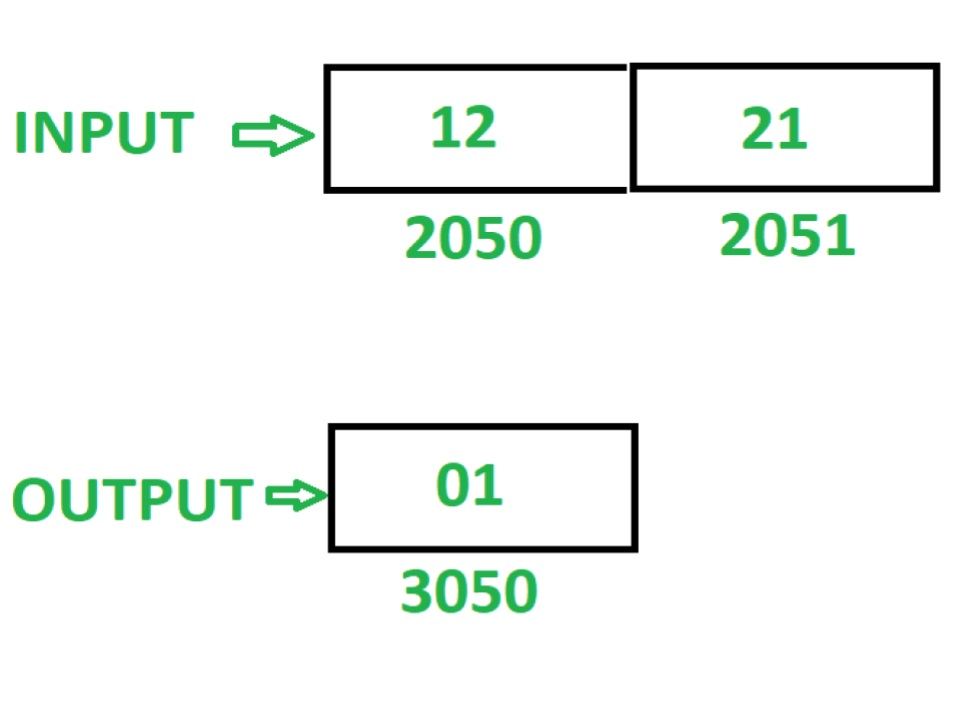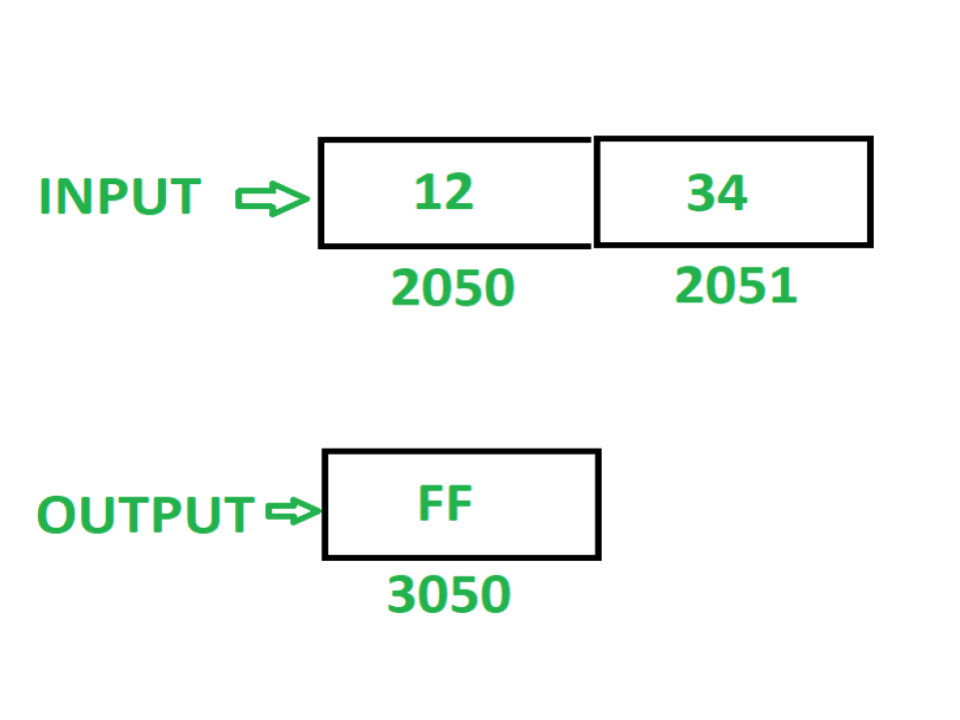Open in App
Not now

# 8085 program to check whether the given 16 bit number is palindrome or not

• Difficulty Level : Basic
• Last Updated : 31 May, 2018

Problem – Write an assembly language program to check whether the given 16 bit number is palindrome or not. If number is palindrome then store 01 at memory location 3050 otherwise store FF at memory location 3050.

Note – A palindrome number is a number that remains the same when its digits are reversed.

Assume that 16 bit number, to check for palindrome is stored at memory location 2050.

Examples –Algorithm –

1. Load contents of memory location 2050 in register L and contents of memory location 2051 in register H
2. Move contents of L in accumulator A
3. Reverse the contents of A by executing RLC instruction 4 times
4. Move the contents of A in L
5. Move the contents of H in A
6. Reverse the contents of A by executing RLC instruction 4 times
7. Move the contents of L in H
8. Move the contents of A in L
9. Store the content of L in memory location 2070 and contents of H in memory location 2071
10. Load the content of memory location 2050 in A
11. Move the content of A in register B
12. Load the content of memory location 2070 in A
13. Compare content of A and B. If the content is not same then store FF in A and store it in memory location 3050
14. If contents of A and B are same, then Load the content of memory location 2051 in A
15. Move the content of A in B
16. Load the content of memory location 2071 in A
17. Compare content of A and B. If the content is not same then store FF in A and store it in memory location 3050
18. If contents of A and B are same, then store 01 in A and store it in memory location 3050

Program –

2000 LHLD 2050 L <- M, H <- M
2003 MOV A, L A <- L
2004 RLC Rotate accumulator content left by 1 bit without carry
2005 RLC Rotate accumulator content left by 1 bit without carry
2006 RLC Rotate accumulator content left by 1 bit without carry
2007 RLC Rotate accumulator content left by 1 bit without carry
2008 MOV L, A L <- A
2009 MOV A, H A <- H
200A RLC Rotate accumulator content left by 1 bit without carry
200B RLC Rotate accumulator content left by 1 bit without carry
200C RLC Rotate accumulator content left by 1 bit without carry
200D RLC Rotate accumulator content left by 1 bit without carry
200E MOV H, L H <- L
200F MOV L, A L <- A
2010 SHLD 2070 M <- L, M <- H
2013 LDA 2050 A <- M
2016 MOV B, A B <- A
2017 LDA 2070 A <- M
201A CMP B A – B
201B JZ 2024 Jump if ZF = 0
201E MVI A, FF A <- 01
2020 STA 3050 M <- A
2023 HLT END
2024 LDA 2051 A <- M
2027 MOV B, A B <- A
2028 LDA 2071 A <- M
202B CMP B A – B
202C JZ 2035 Jump if ZF = 0
202F MVI A, FF A <- FF
2031 STA 3050 M <- A
2034 HLT END
2035 MVI A, 01 A <- 01
2037 STA 3050 M <- A
203A HLT END

Explanation – Registers A, H, L, B are used for general purpose.

1. LHLD 2050: loads contents of memory location 2050 in L and 2051 in H.
2. MOV A, L: moves content of L in A.
3. RLC: shift the content of A left by one bit without carry. Repeat the current instruction 4 times so that contents of A get reversed.
4. MOV L, A: moves the content of A in L.
5. MOV A, H: moves the content of H in A.
6. RLC: shift the content of A left by one bit without carry. Repeat the current instruction 4 times so that contents of A get reversed.
7. MOV H, L: moves the content of L in H.
8. MOV L, A: moves the content of A in L.
9. SHLD 2070: stores the content of L in 2070 and H in 2071.
10. LDA 2050: load the content of memory location 2050 in A.
11. MOV B, A: moves the content of A in B.
12. CMP B: compares the content of A and B. It set the zero flag if content is same otherwise reset.
13. JZ 2024: jump to memory location 2024 if ZF = 1.
14. MVI A, FF: store FF in A.
15. STA 3050: store content of A in 3050.
16. HLT: stops executing the program and halts any further execution.
17. LDA 2051: load the content of memory location 2050 in A.
18. MOV B, A: moves the content of A in B.
19. LDA 2071: load the content of memory location 2071 in A.
20. CMP B: compares the content of A and B. It set the zero flag if content is same otherwise reset.
21. JZ 2035: jump to memory location 2035 if ZF = 1.
22. MVI A, FF: store FF in A.
23. STA 3050: store content of A in 3050.
24. HLT: stops executing the program and halts any further execution.
25. MVI A, 01: store 01 in A.
26. STA 3050: store content of A in 3050.
27. HLT: stops executing the program and halts any further execution.

My Personal Notes arrow_drop_up
Related Articles